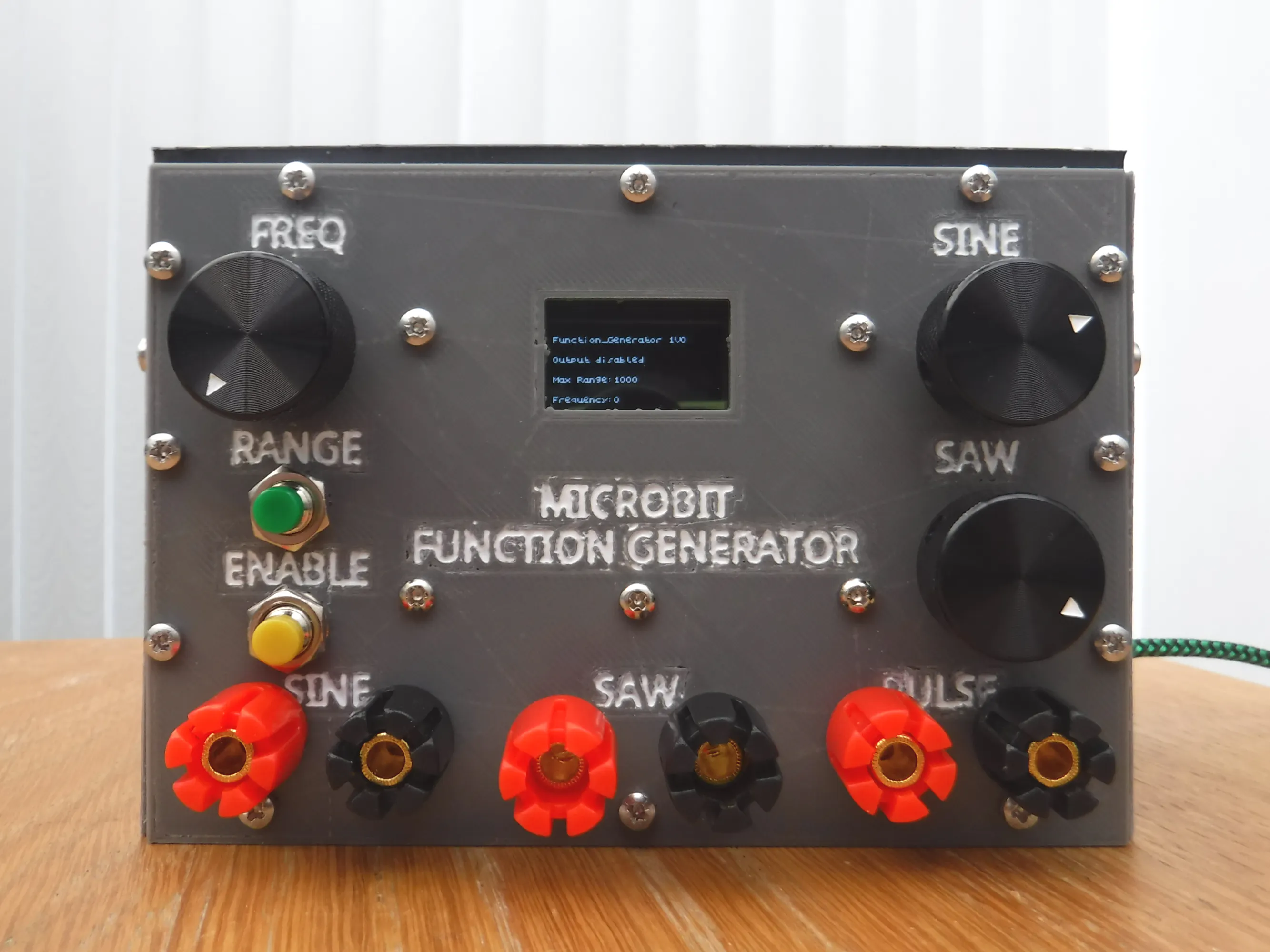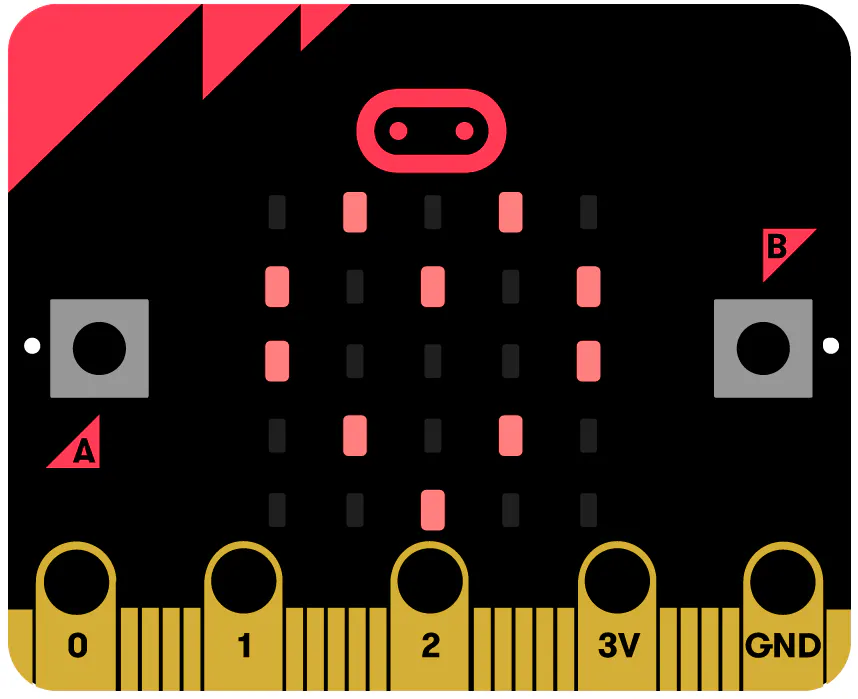Created October 18, 2021 © CC BY-NC-SA

Microbit Function Generator

A Function generator producing sine, sawtooth and pulses up to 1MHz using a Microbit, custom PCB and 3d printed box.Things used in this project

Hardware componentsBBC micro:bit board
×1
 OLED Display
×1Test Accessory, Dual Banana Jack to Male BNC Adapter Optional connections
×3
 5K Potentiometer
×2
 100k Multiturn Potentiometer
×1
 Control Knobs 20 x 15.5 mm, ~6mm diameter spindle Subject to personal choice and potentiometer selection.
×3
 Microbit breakout board
×1
 Jumper wires M/F
×23
 Resistor 15k
×3
 Resistor 30k
×3
 Resistor 60k
×3
 Resistor 120k
×3
 Resistor 100k
×1
 Resistor 200R
×2
 Resistor 150R
×1
 Resistor 330R
×1
 Capacitor 100nF
×6
 Capacitor 100uF/10V
×1
 CD4024 - 7 stage Binary Counter
×1
 CD4015 - Dual shift register
×1
 CD4070 - Quad 2 input EXOR
×1
 MCP602 - Dual Op-Amp
×1
 Terminal Block - 2 pin 5mm pitch
×1
 M3 8mm screw
×32
 M3 10mm screw
×1
 M3 30mm screw
×2

Software apps and online services

 Microbit makecode
 Slicer Cura

Hand tools and fabrication machines3D Printer (generic)Soldering iron (generic)Wire Stripper & Cutter, 26-16 AWG / 0.4-1.29mm Capacity Wires

Custom parts and enclosures

Enclosure STL files

Files required to 3d printing the side panela

Schematics

D2A converter

PCB gerber files

Files to enable PCB creation

Code

microbit_VPEN_VIEW_JS.txt

JavaScript
Microbit code for Function Generator (Makecode conversion)
function centre () {
if (o_enable == 0) {
led.unplot(2, 2)
led.plot(4, 4 - range)
} else {
led.plot(2, 2)
led.plot(4, 4 - range)
}
}
input.onButtonPressed(Button.A, function () {
if (o_enable == 0) {
o_enable = 1
old = 0
led.plot(2, 2)
} else {
o_enable = 0
led.unplot(2, 2)
}
if (o_enable == 0) {
kitronik_VIEW128x64.show("Output disabled", 3)
} else {
kitronik_VIEW128x64.show("Output enabled", 3)
}
})
freq = 0
pct = (bin - 1) / 1023 + offset[range]
if (pct > 0) {
freq = max_range * pct
}
if (old > bin - 20 && old < bin + 20) {
clr_scrn()
for (let index2 = 0; index2 <= 9 * (pct * 1.25); index2++) {
indx2 = -4 + index2
for (let index = 0; index <= 4; index++) {

}
}
kitronik_VIEW128x64.show("Frequency:" + Math.trunc(freq) + "  ", 7)
}
old = bin
centre()
}
function clr_scrn () {
for (let index2 = 0; index2 <= 9 * (1 * 1.25); index2++) {
indx2 = -4 + index2
for (let index = 0; index <= 4; index++) {
led.unplot(index, index - indx2)
}
}
centre()
}
input.onButtonPressed(Button.B, function () {
range += 1
if (range > list.length - 1) {
range = 0
led.unplot(4, 0)
led.plot(4, 4)
} else {
led.unplot(4, 4 - (range - 1))
led.plot(4, 4 - range)
}
max_range = list[range]
kitronik_VIEW128x64.show("Max Range:" + list[range] + "  ", 5)
})
function reset () {
list = [
1000,
10000,
100000,
250000,
500000
]
max_range = list
offset = [
0.007,
0.0069,
0.00685,
0.006844,
0.005906
]
xval = 0
yval = -1
value = -1
xsig = 3
ysig = -1
scale = -1
freq = 0
range = 0
multiplier = 0
o_enable = 0
pins.analogPitch(0, 0)
music.ringTone(0)
}
let multiplier = 0
let scale = 0
let ysig = 0
let xsig = 0
let value = 0
let yval = 0
let xval = 0
let indx2 = 0
let max_range = 0
let offset: number[] = []
let pct = 0
let bin = 0
let old = 0
let range = 0
let o_enable = 0
let freq = 0
let list: number[] = []
pins.analogSetPitchPin(AnalogPin.P0)
music.setBuiltInSpeakerEnabled(false)
basic.showString("F_GEN")
reset()
led.plot(4, 4)
kitronik_VIEW128x64.controlDisplayOnOff(kitronik_VIEW128x64.onOff(true))
kitronik_VIEW128x64.show("Function_Generator 1V0", 1)
kitronik_VIEW128x64.show("Output disabled", 3)
kitronik_VIEW128x64.show("Max Range:" + list, 5)
kitronik_VIEW128x64.show("Frequency:" + Math.trunc(freq), 7)
basic.forever(function () {
if (o_enable == 1) {
if (freq >= 1) {
music.ringTone(freq)
} else {
music.ringTone(0)
}
} else {
music.ringTone(0)
}
})

Credits

C Forde

2 projects • 0 followers